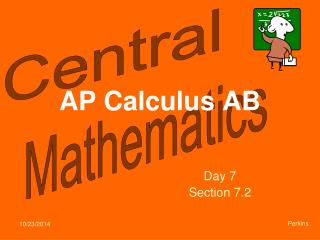DownloadDownload PresentationAP Calculus AB

# AP Calculus AB

Télécharger la présentation## AP Calculus AB

- - - - - - - - - - - - - - - - - - - - - - - - - - - E N D - - - - - - - - - - - - - - - - - - - - - - - - - - -
##### Presentation Transcript

1. AP Calculus AB Day 7 Section 7.2 Perkins

2. Theorem: The volume of a solid with cross-section of area A(x) that is perpendicular to the x-axis is given by ***This circle is the base of a 3-D figure coming out of the screen. S a b ***This rectangle is a side of a geometric figure (a cross-section of the whole). Finding the volume of a solid with known cross-section is a 3-step process: Step #1: Find the length (S) of the rectangle used to create the base of the figure. Step #2: Find the area A(x) of each cross-section (in terms of this rectangle). Step #3: Integrate the area function from the lower to the upper bound. Volume of a solid with cross-sections of area A(y) and perpendicular to the y-axis: d S c

3. Find the volume of the solid whose base is bounded by the circle with cross-sections perpendicular to the x-axis. These cross-sections are a. squares Step #1: Find S. S -2 2 Step #2: Find A(x). S Step #3: Integrate the area function.

4. Find the volume of the solid whose base is bounded by the circle with cross-sections perpendicular to the x-axis. These cross-sections are b. equilateral triangles Step #1: Find S. S -2 2 Step #2: Find A(x). S Step #3: Integrate.

5. AP Calculus AB Day 7 Section 7.2 Perkins

6. Theorem: The volume of a solid with cross-section of area A(x) that is perpendicular to the x-axis is given by a b Finding the volume of a solid with known cross-section is a 3-step process:

7. Find the volume of the solid whose base is bounded by the circle with cross-sections perpendicular to the x-axis. These cross-sections are a. squares Step #1: Find S. Step #2: Find A(x). Step #3: Integrate the area function.

8. Find the volume of the solid whose base is bounded by the circle with cross-sections perpendicular to the x-axis. These cross-sections are b. equilateral triangles Step #1: Find S. Step #2: Find A(x). Step #3: Integrate.